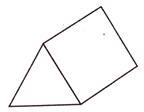# Directions: Consider the figure given below:Based on the figure, answer the following questions:Q. The number of edges in the figure is _________a)4                     b)6           c)8                     d)9e)None of theseCorrect answer is option 'D'. Can you explain this answer? Related Test: Test: Shapes (Geometry) - 3

## Class 3 QuestionAnshuman Kumar Jul 28, 2020
Answer is dRamana Chintayala Aug 15, 2020
9Amrita Nov 24, 2020
6RAJ NISHA Jun 11, 2020
The correct answer is d
The number of edges in the figure is 9.Mohammad Khan Dec 18, 2020
SIXSankar Saha Jul 24, 2020
Answer is 9Taapasya Bhat Sep 10, 2020
D as it is 9Sonia Bhat Jun 12, 2020
DKahna Oct 16, 2020
B.6Shams Khan Aug 15, 2020
No, the correct answer is e ( none of these) because there are two triangles and two rectangles but if we will count two triangles then only 4 sides will be left this means that if we count the edges they will be equal to 10 sides.Rakesh Kasaudhan Kasaudh Jan 09, 2021
Ans d 9Adarsh Kumar Jan 30, 2021
Option bBad Gamer Sep 17, 2020
BSwapnil Roy Oct 07, 2020
Answer is 6Shivaji Waghmare Feb 28, 2021
या 4Iam Genius Sep 06, 2020
D is the correct answer

This discussion on Directions: Consider the figure given below:Based on the figure, answer the following questions:Q. The number of edges in the figure is _________a)4 b)6c)8 d)9e)None of theseCorrect answer is option 'D'. Can you explain this answer? is done on EduRev Study Group by Class 3 Students. The Questions and Answers of Directions: Consider the figure given below:Based on the figure, answer the following questions:Q. The number of edges in the figure is _________a)4 b)6c)8 d)9e)None of theseCorrect answer is option 'D'. Can you explain this answer? are solved by group of students and teacher of Class 3, which is also the largest student community of Class 3. If the answer is not available please wait for a while and a community member will probably answer this soon. You can study other questions, MCQs, videos and tests for Class 3 on EduRev and even discuss your questions like Directions: Consider the figure given below:Based on the figure, answer the following questions:Q. The number of edges in the figure is _________a)4 b)6c)8 d)9e)None of theseCorrect answer is option 'D'. Can you explain this answer? over here on EduRev! Apart from being the largest Class 3 community, EduRev has the largest solved Question bank for Class 3.